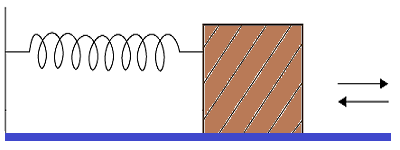## Thursday, November 4, 2021

### Problems 2: UNIZOR.COM - Physics4Teens - Waves - Mechanical Oscillations

Notes to a video lecture on http://www.unizor.com

Problems 2

Problem AAn object of mass m=0.3 kg sits on a table and is attached to a wall by a horizontal spring with elasticity κ=2 N/m.
This object can slide left and right on a surface of a table with the static and dynamic friction coefficient μ=0.1.
Initially, the spring is in equilibrium position, not stretched, not squeezed.
What is the maximum distance λ we can stretch a spring such that the object would remain in that position on a stretched spring and not moved back towards equilibrium?
Assume that gravity acceleration is g=9.8 m/sec².

Solution

The necessary condition for an object to remain in place with a stretched spring is that the force of a spring that pulls the object back to equilibrium Fs is not sufficient to overcome the static friction force Ff.
Fs = κ·d = 2·d (N)
Ff = μ·m·g = 0.1·0.3·9.8 (N)
The necessary condition for an object to remain in place on a stretched spring is
Fs ≤ Ff
That is,
2(N/m)·λ(m) ≤ 0.1·0.3·9.8 (N)
from which follows
λ ≤ 0.147 (m)

The maximum distance to stretch a spring with an object not being pulled back (critical distance) is
λ = 0.147m = 147mm

Problem B

Consider the same set-up as in the Problem A.
Our task now is to stretch a spring beyond the critical distance λ, so that the object will be pulled back by a spring, but it should reach the equilibrium point and stop there with no further movements.
What is the distance d to stretch a spring to achieve this result?

Solution

Let's approach this problem from the energy standpoint.
As we know, the potential energy of a spring stretched by a distance d is
U(d) = κ·d²/2
(see "Energy of Oscillation" lecture in this chapter of a course)
Using the data from Problem A,
U(d) = 2·d²/2
This energy should be fully used to overcome the constant friction force Ff on a distance d.
Ff = μ·m·g = 0.294 (N)
On a distance d the force Ff performs work
W(d) = Ff·d = 0.294·d
which should be equal to initial potential energy U(d).
Therefore,
W(d) = U(d)
0.294·d = 2·d²/2
d = 0.294 (m)